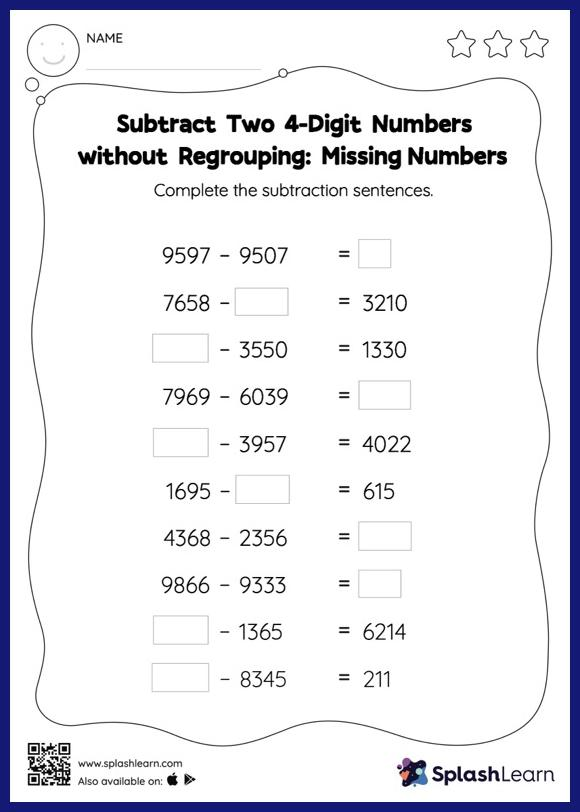# Subtract Two 4-Digit Numbers without Regrouping: Missing Numbers Worksheet

Home > Subtract Two 4-Digit Numbers without Regrouping: Missing NumbersIn this worksheet, students must find the missing number using the count back method or the relationship between addition and subtraction. To solve subtract two 4-digit numbers without regrouping worksheet, they do not need to regroup the numbers. In this worksheet, students practice solving problems written in the horizontal format. How numbers are laid out in a problem affects the method a student employs to solve it. Therefore to develop actual fluency and mastery of multiple strategies, students must practice different formats.